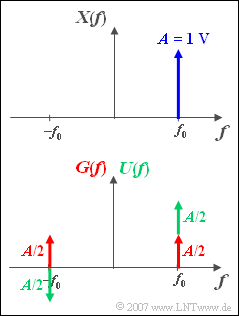# Exercise 3.6Z: Complex Exponential FunctionSplitting the complex exponential function in the spectral domain

In connection with  "band-pass systems" , one-sided spectra are often used.  In the graphic you see such a one-sided spectral function  ${X(f)}$, which results in a complex time signal  ${x(t)}$.

In the sketch below,  ${X(f)}$  is split into an even component  ${G(f)}$  – with respect to the frequency – and an odd component  ${U(f)}$.

Hints:

### Questions

1

What is the time function  $g(t)$  that fits  $G(f)$?  How large is   $g(t = 1 \, µ \text {s})$?

 $\text{Re}\big[g(t = 1 \, µ \text {s})\big] \ = \$  $\text{V}$ $\text{Im}\big[g(t = 1 \, µ \text {s})\big]\ = \$  $\text{V}$

2

What is the time function  $u(t)$  that fits  $U(f)$?  What is the value of  $u(t = 1 \, µ \text {s})$?

 $\text{Re}\big[u(t = 1 \, µ \text {s})\big]\ = \$  $\text{V}$ $\text{Im}\big[u(t = 1 \, µ \text {s})\big]\ = \$  $\text{V}$

3

Which of the statements are true regarding the signal  $x(t)$ ?

 The signal is  $x(t) = A \cdot {\rm e}^{{\rm j}\hspace{0.05cm}\cdot \hspace{0.05cm}2\pi\hspace{0.05cm}\cdot \hspace{0.05cm} f_0 \hspace{0.05cm}\cdot \hspace{0.05cm}t}$. In the complex plane  $x(t)$  rotates clockwise. In the complex plane  $x(t)$  rotates counterclockwise. One microsecond is needed for one rotation.

### Solution

#### Solution

(1)  $G(f)$  is the spectral function of a cosine signal with period  $T_0 = 1/f_0 = 8 \, µ\text {s}$:

$$g( t ) = A \cdot \cos ( {2{\rm{\pi }}f_0 t} ).$$

At  $t = 1 \, µ\text {s}$  the signal value is equal to  $A \cdot \cos(\pi /4)$:

• The real part is  $\text{Re}[g(t = 1 \, µ \text {s})] = \;\underline{0.707\, \text{V}}$,
• The imaginary part is  $\text{Im}[g(t = 1 \, µ \text {s})] = \;\underline{0.}$

(2)  Starting from the Fourier correspondence

$$A \cdot {\rm \delta} ( f )\ \ \circ\!\!-\!\!\!-\!\!\!-\!\!\bullet\, \ \ A$$

is obtained by applying the shifting theorem twice (in the frequency domain):

$$U( f ) = {A}/{2} \cdot \delta ( {f - f_0 } ) - {A}/{2} \cdot \delta ( {f + f_0 } )\ \ \circ\!\!-\!\!\!-\!\!\!-\!\!\bullet\, \ \ u( t ) = {A}/{2} \cdot \left( {{\rm{e}}^{{\rm{j}}\hspace{0.05cm}\cdot \hspace{0.05cm}2{\rm{\pi }}\hspace{0.05cm}\cdot \hspace{0.05cm}f_0\hspace{0.05cm}\cdot \hspace{0.05cm} t} - {\rm{e}}^{{\rm{ - j}}\hspace{0.05cm}\cdot \hspace{0.05cm}2{\rm{\pi }}\hspace{0.05cm}\cdot \hspace{0.05cm}f_0 \hspace{0.05cm}\cdot \hspace{0.05cm}t} } \right).$$
$$u( t ) = {\rm{j}} \cdot A \cdot \sin ( {2{\rm{\pi }}f_0 t} ).$$
• The real part of this signal is always zero.
• At  $t = 1 \, µ\text {s}$  the following applies to the imaginary part:  $\text{Im}[g(t = 1 \, µ \text {s})] = \;\underline{0.707\, \text{V}}$.

(3)  Because  $X(f) = G(f) + U(f)$  also holds:

$$x(t) = g(t) + u(t) = A \cdot \cos ( {2{\rm{\pi }}f_0 t} ) + {\rm{j}} \cdot A \cdot \sin( {2{\rm{\pi }}f_0 t} ).$$

This result can be summarised by  "Euler's theorem"  as follows:

$$x(t) = A \cdot {\rm{e}}^{{\rm{j}}\hspace{0.05cm}\cdot \hspace{0.05cm}2{\rm{\pi }}\hspace{0.05cm}\cdot \hspace{0.05cm}f_0 \hspace{0.05cm}\cdot \hspace{0.05cm}t} .$$

The given alternatives 1 and 3 are correct:

• The signal rotates in the complex plane in a mathematically positive direction, i.e. counterclockwise.
• For one rotation, the "pointer" needs the period  $T_0 = 1/f_0 = 8 \, µ\text {s}$.